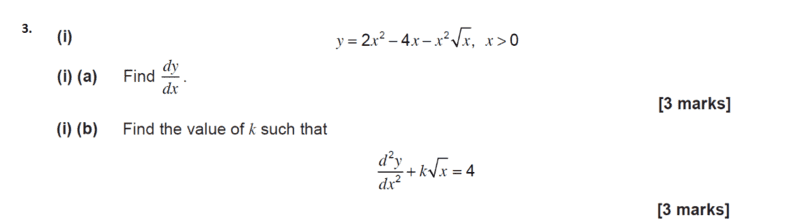# Solving an equation with a parameter and a derivative

• I
• homeworkhelpls

#### homeworkhelpls

TL;DR Summary
For 3(i)(b) does anyone know how to find the value of k?idk how to start after finding the second derivative

#### Attachments

You have
$$\frac{d^2y}{dx^2} = 4 - \frac{15}{4} \sqrt{x}$$
You can either rearrange that equation so that it looks like
$$\frac{d^2y}{dx^2} + k \sqrt{x} = 4$$
and identify what ##k## is, or use a more robust approach by substituting the ##\frac{d^2y}{dx^2}## you have found into that second equation,
$$\left(4 - \frac{15}{4} \sqrt{x} \right) + k \sqrt{x} = 4$$
and solve for ##k##.

Thread closed. The OP has been warned five times previously that homework-type questions must be posted in one of the forum sections devoted to homework questions.